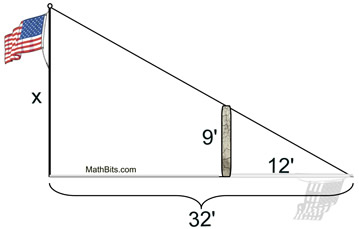Practice with Similarity Terms of Use    Contact Person: Donna Roberts1.
Rhombus ABCD and rhombus EFGH are similar with a ratio of similitude of 5 : 2. Find x.

Choose:
 2 3 5 6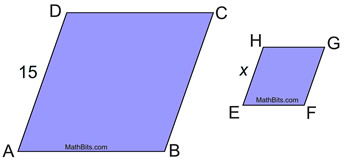with four equal sides.

2.
ΔCAT and ΔDOG are shown at the right. You are told that mA and mO are each 58º.
Find DO.

Choose:
 8 10 12 18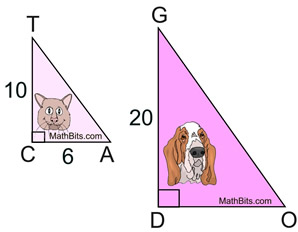3.
Which of the following triangles are always similar?

Choose:
 right triangles isosceles triangles equilateral triangles acute triangles4.
Given the overlapping triangles shown at the right. Notice the parallel segments.
Find x.

Choose:
 6 8 12 15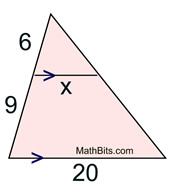5.
Given: These two regular polygons are similar. What is their ratio of similitude (from larger to smaller)?

Choose:
 12 : 8 2 : 1 4 : 8 8 : 2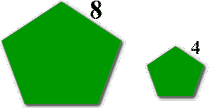A regular polygon has all of its sides of equal length and all of its angles of equal measure.

6.
Two triangle roofs are similar. The corresponding sides of these roofs are 16 feet and 24 feet. If the altitude of the smaller roof is 6 feet, find the corresponding altitude of the larger roof.

Choose:
 9 10 11 12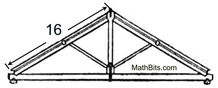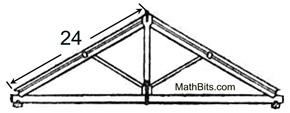7.
In ΔABC, m∠A = 90º and m∠B = 35º.
In ΔDEF, m∠E = 35º and m∠F = 55º.
a) Are these triangles similar?
Explain.
Choose:
 YES NOb)
If AB = 30, AC = 40, and DE = 24, what is the length of DF?

Choose:
 26 28 30 32

8.
Given the diagram at the right with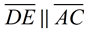.
Find BC.

Choose:
 4 4.5 13.5 18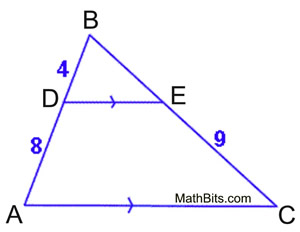9.
ΔABC is similar to ΔDEF as shown. The shortest side of the larger triangle is 21. What is the perimeter of the larger triangle, ΔABC?
Choose:
 27 36 63 81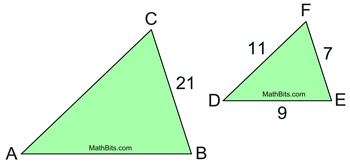10. The legs of right ΔABC are 2.8 inches and 1.4 inches. If right ΔDEF is similar to ΔABC, which of the following lengths could represent the legs of ΔDEF? (Choose all that apply and hit SUBMIT!)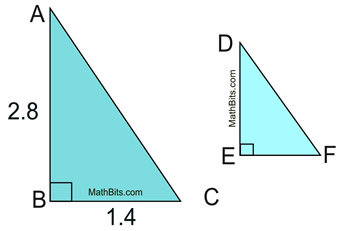Diagrams are not drawn to scale. 2 inches and 1 inch 1 inch and 0.75 inches 2.2 inches and 1.2 inches 1.25 inches and 0.625 inches 0.85 inches and 0.45 inches

 11 Given the diagram shown at the right with parallel lines indicated. a) Explain why ΔACB is similar to ΔDCE Explanation By alternate interior angles, angle BAC congruent to angle DEC. angle ABC congruent to angle CED. (also vertical angles at C) This gives AA for similar triangles b) If m∠ACE = 112º, find m∠ABC + m∠CDE. Explain your answer. Explanation Angle ACE is an exterior angle. Exterior angle = sum nonadjacent interior angles. m(angle)ABC + m(angle)CDE = 112º.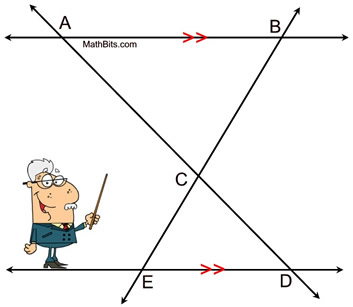12.
A vertical flagpole casts a shadow 32 feet long at the same time that a nearby vertical 9 foot post casts a shadow 12 feet long. What is the height of the flagpole in feet?
Choose:
 22 feet 24 feet 26 feet 28 feet